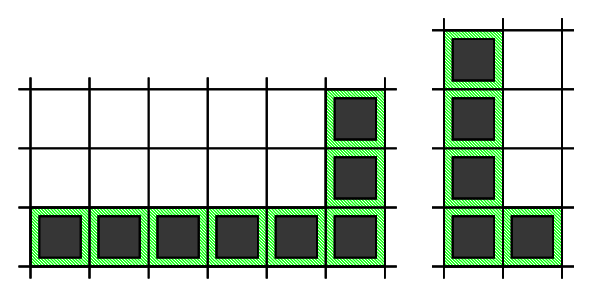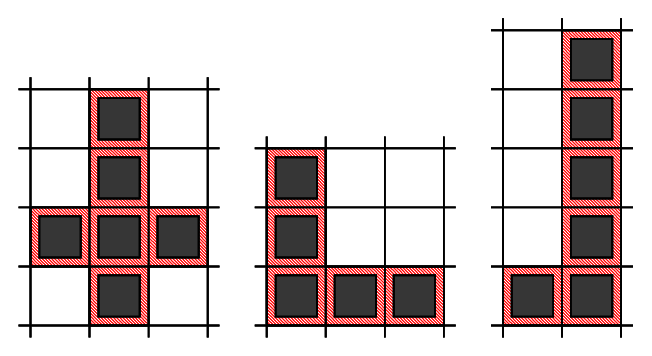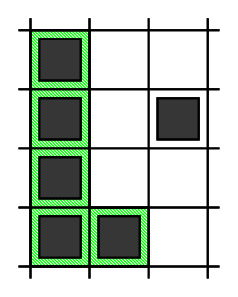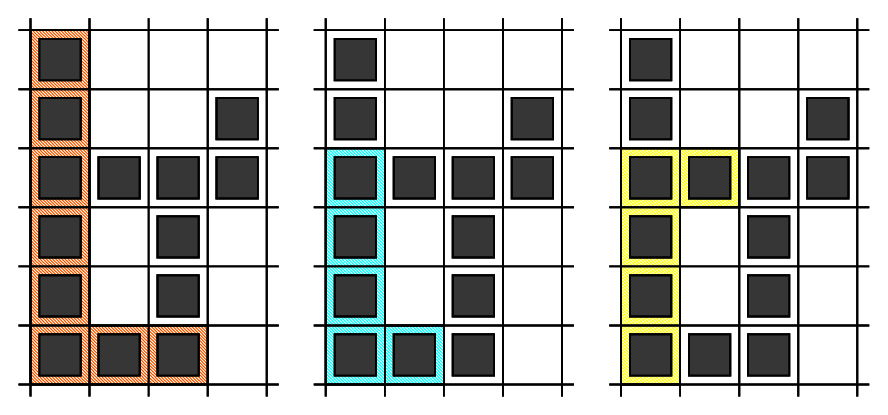시간 제한 메모리 제한 제출 정답 맞은 사람 정답 비율
60 초 (추가 시간 없음) 1024 MB (추가 메모리 없음) 7 7 7 100.000%

## 문제

Given a grid of R rows and C columns each cell in the grid is either 0 or 1.

A segment is a nonempty sequence of consecutive cells such that all cells are in the same row or the same column. We define the length of a segment as number of cells in the sequence.

A segment is called "good" if all the cells in the segment contain only 1s.

An "L-shape" is defined as an unordered pair of segments, which has all the following properties:

• Each of the segments must be a "good" segment.
• The two segments must be perpendicular to each other.
• The segments must share one cell that is an endpoint of both segments.
• Segments must have length at least 2.
• The length of the longer segment is twice the length of the shorter segment.

We need to count the number of L-shapes in the grid.

Below you can find two examples of correct L-shapes,and three examples of invalid L-shapes.Note that in the shape on the left, two segments do not share a common endpoint. The next two shapes do not meet the last requirement, as in the middle shape both segments have the same length, and in the last shape the longer segment is longer than twice the length of the shorter one.

## 입력

The first line of the input contains the number of test cases, T. T test cases follow.

The first line of each testcase contains two integers R and C.

Then, R lines follow, each line with C integers representing the cells of the grid.

## 출력

For each test case, output one line containing Case #x: y, where x is the test case number (starting from 1) and y is the number of L-shapes.

## 제한

• 1 ≤ T ≤ 100.
• Grid consists of 0s and 1s only.

• 1 ≤ R ≤ 40.
• 1 ≤ C ≤ 40.

## Test Set 2 (12점)

• 1 ≤ R ≤ 1000 and 1 ≤ C ≤ 1000 for at most 5 test cases.
• For the remaining cases, 1 ≤ R ≤ 40 and 1 ≤ C ≤ 40.

## 예제 입력 1

2
4 3
1 0 0
1 0 1
1 0 0
1 1 0
6 4
1 0 0 0
1 0 0 1
1 1 1 1
1 0 1 0
1 0 1 0
1 1 1 0


## 예제 출력 1

Case #1: 1
Case #2: 9


## 힌트

In Sample Case #1, there is one L-shape.

• The first one is formed by using cells: (1,1), (2,1), (3,1), (4,1), (4,2)In Sample Case #2, there are nine L-shapes.

• The first one is formed by using cells: (1,1), (2,1), (3,1), (4,1), (5,1), (6,1), (6,2), (6,3)
• The second one is formed by using cells: (3,1), (4,1), (5,1), (6,1), (6,2)
• The third one is formed by using cells: (6,1), (5,1), (4,1), (3,1), (3,2)
• The fourth one is formed by using cells: (3,3), (4,3), (5,3), (6,3), (6,2)
• The fifth one is formed by using cells: (6,3), (5,3), (4,3), (3,3), (3,2)
• The sixth one is formed by using cells: (3,1), (3,2), (3,3), (3,4), (2,4)
• The seventh one is formed by using cells: (3,4), (3,3), (3,2), (3,1), (2,1)
• The eighth one is formed by using cells: (3,4), (3,3), (3,2), (3,1), (4,1)
• The ninth one is formed by using cells: (6,3), (5,3), (4,3), (3,3), (3,4)

The first three L-shapes are shown on the picture below.## 채점 및 기타 정보

• 예제는 채점하지 않는다.